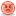##I need help with this program!!

I have a program that I need to modify but I am stuck. Here are the terms.

- Modify the program by inserting a big part into a loop that will play the program over and over and will display a number of times you have played the game.

- Have the program ask you how many times you want to play the game.
- Then the program will play the game the number of times you request.
- Keeping track of the number of times there was a win or loss.
- Have the program display the percent (%) of times there was a win.

Here is the sample program that I have to go off to include these stipulations above.

#include <iostream>
#include <cstdlib>
#include <ctime>

using namespace std;

int rolldice ();

void main ()
{
enum Status {CONTINUE, WON, LOST};
int Sum, MyPoint;
Status GameStatus;

srand(time(0));

//Roll the dice
Sum = rolldice();
cout << "You rolled " << Sum << '.' << endl;

switch (Sum)
{
case 7:
case 11:
GameStatus = WON;
break;
case 2:
case 3:
case 12:
GameStatus = LOST;
break;
default:
GameStatus = CONTINUE;
MyPoint = Sum;
cout << "Point is " << MyPoint << '.' << endl;
break;
}

while (GameStatus == CONTINUE)
{
Sum = rolldice();
cout << "The roll is " << Sum << '.' << endl;
if (Sum == MyPoint)
GameStatus = WON;
else
if (Sum == 7)
GameStatus = LOST;

}

if (GameStatus == WON)
cout << "You win the game." << endl;
else
cout << "You lose the game." << endl;
}

int rolldice ()
{
int Die1, Die2;
int Total;
Die1 = (rand() % 6) +1;
Die2 = (rand() % 6) +1;
Total = Die1 + Die2;